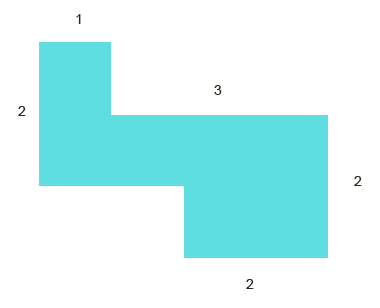# Numb3rs 320: Burn Rate

In this episode the team investigates a series of bombings. By looking at where the bombings occurred, Charlie is able to guess where the bomber lives.

## CentroidsIn the show Charlie plots the bombing locations on a map and is able to the 'center' of those points, which is the most likely place the bomber would chose as a base of operations. There are many geometric notions of center of a collection of points in the plane. One obvious one is the point which minimizes the sums of distance from that point to all others. Unfortunately, such a point is often difficult or impossible to find explicitly. This is because the distance between two points involves a square roots which are difficult functions to analyze.

Instead of minimizing the distance to find the center we might try minimizing the square of the distance. This eliminates problem-causing square roots. This method is called ßleast squares and is a very common method for fitting models to data. Applications range from basic data analysis like you might do in a physics or chemistry class to making noise canceling headphones or guiding ballistic missiles.

A different and fruitful notion of center is that of the centroid. You might be familiar with this concept from geometry. For a triangle the centroid is the intersection of the median lines, and in general the centroid of a regular polygon is the center of the polygon. Formally, for n points in the plane the centroid, (Cx, Cy), is the average of the coordinates of the points. That is Cx=&Sigmai=1nxi/n, and Cy=&Sigmai=1nyi/n. From the definition we can see that we can still find the centroid even if the points don't define a regular polygon, or even a polygon at all.

Activity 1
1. 1. For a regular hexagon show that the definition given above for the centroid agrees with what you would consider to be the center of the hexagon
2. 2. Show that the centroid corresponds to the center of a regular polygon with n sides (Hint: think about the symmetries of the polygon).

### Centers of Mass

We can expand the idea of the centroid in two ways. One is to gives each point a mass mi. Then the centroid is given by Cx=&Sigmai=1nmixi/n, and Cy=&Sigmai=1nmiyi/n. If you imagine connecting these points to each other and the centroid using mass-less, inflexible wires, then you would be able to balance this object by placing your finger underneath the centroid. Thus this centroid corresponds to the center of mass of the points. Note that for regular polygons we treat all the points as having mass 1.

Activity 2: Is the center of mass of a planar object of uniform density always contained within the object? Can you say which kind of object always contains its center of mass (Hint: you may have heard of this term in a geometry class or used to describe curves)?

The second generalization is to move away from points to contiguous objects. Since we now have infinitely many points we need to suppose that the object has some density &delta so that a single point weighs nothing, but groups of them have some mass. The centroid is then given by Cx=(&int &int x &delta dA)/M and Cy=(&int &int y &delta dA)/M, where M is the total mass of the object. If M is not known it can be calculated as M=&int &int &delta dA. The double integral and dA indicate that we are integrating over the area of the object. If you recall that integrals are just limits of sums and the density is just the amount of mass per area you can see how this definition is related to those given above. In greater generality we can allow the density of our object to vary as a function of x and y and another integral to find the center of mass of 3-dimensional objects with variable density. Thus with enough patience you would use this method to find the center of mass of anything in the world.

Activity 3:

You can often approximate the center of mass of an object with uniform density without resorting to integration. The first step is to realize that you already know the center of mass of some simple shapes like filled in regular polygons as their centers of mass correspond to the centroids of regular polygons in the plane. Next you break your object down into polygons and find the centroids of these polygons. Now you've reduced to problem to finding the center of mass of the centroids where each centroid has mass equal to area of its polygon times the density of the object.

Try this for the figure below. Assume that the density is 1.